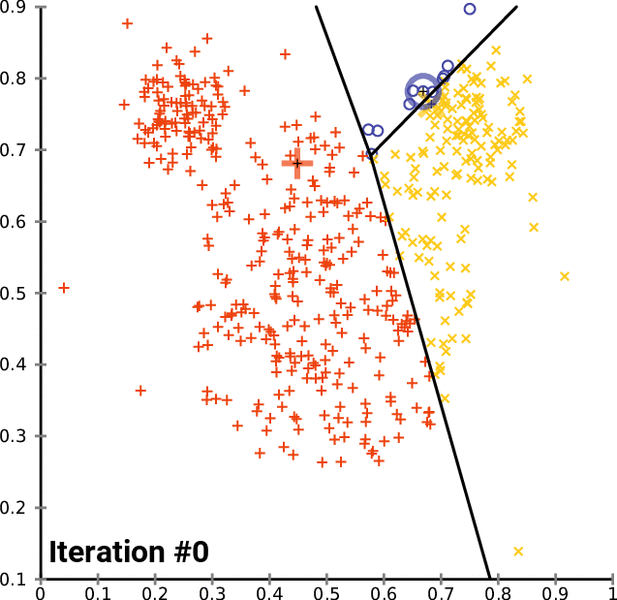# How to program the K-Means clustering algorithm

K-Means is a popular unsupervised machine learning algorithm for data clustering. A typical start for flat clustering, the K-Means algorithm works by defining a number K of clusters to be extracted by the algorithm. With this K number given, the algorithm will then find the best “centroids” to cluster the data around.To go into the details of K-Means and to program it in Python, this complete series of tutorials by Harrison Kinsley, a.k.a. Sentdex, dives in all the details of the K-Means clustering algorithm, programming tricks and present example uses.

The final code can also be obtained from Sentdex Python Programming website, in the corresponding K-Means tutorial with more examples, a comparison with the “Titanic dataset”, details on the code and links to other key concepts and Python functions.

The following videos regroup the Support Vector Machine tutorials from the Machine Learning with Python series (parts 34 to 38) by Sentdex on Youtube.

For a complete presentation of this clustering algorithm, with mathematical formulas and extra developments, check the Wikipedia page on the K-Means clustering algorithm.

For an interesting application, also check this other post on how to find the dominant colors in an image with K-means.

``````
import matplotlib.pyplot as plt
from matplotlib import style
import numpy as np
from sklearn import preprocessing, cross_validation
import pandas as pd

##X = np.array([[1, 2],
## [1.5, 1.8],
## [5, 8],
## [8, 8],
## [1, 0.6],
## [9, 11]])
##
##
##colors = ['r','g','b','c','k','o','y']

class K_Means:
def __init__(self, k=2, tol=0.001, max_iter=300):
self.k = k
self.tol = tol
self.max_iter = max_iter

def fit(self,data):

self.centroids = {}

for i in range(self.k):
self.centroids[i] = data[i]

for i in range(self.max_iter):
self.classifications = {}

for i in range(self.k):
self.classifications[i] = []

for featureset in X:
distances = [np.linalg.norm(featureset-self.centroids[centroid]) for centroid in self.centroids]
classification = distances.index(min(distances))
self.classifications[classification].append(featureset)

prev_centroids = dict(self.centroids)

for classification in self.classifications:
self.centroids[classification] = np.average(self.classifications[classification],axis=0)

optimized = True

for c in self.centroids:
original_centroid = prev_centroids[c]
current_centroid = self.centroids[c]
if np.sum((current_centroid-original_centroid)/original_centroid*100.0) > self.tol:
print(np.sum((current_centroid-original_centroid)/original_centroid*100.0))
optimized = False

if optimized:
break

def predict(self,data):
distances = [np.linalg.norm(data-self.centroids[centroid]) for centroid in self.centroids]
classification = distances.index(min(distances))
return classification

df.drop(['body','name'], 1, inplace=True)
#df.convert_objects(convert_numeric=True)
df.fillna(0,inplace=True)

def handle_non_numerical_data(df):

# handling non-numerical data: must convert.
columns = df.columns.values

for column in columns:
text_digit_vals = {}
def convert_to_int(val):
return text_digit_vals[val]

#print(column,df[column].dtype)
if df[column].dtype != np.int64 and df[column].dtype != np.float64:

column_contents = df[column].values.tolist()
#finding just the uniques
unique_elements = set(column_contents)
# great, found them.
x = 0
for unique in unique_elements:
if unique not in text_digit_vals:
# creating dict that contains new
# id per unique string
text_digit_vals[unique] = x
x+=1
# now we map the new "id" vlaue
# to replace the string.
df[column] = list(map(convert_to_int,df[column]))

return df

df = handle_non_numerical_data(df)

# add/remove features just to see impact they have.
df.drop(['ticket','home.dest'], 1, inplace=True)

X = np.array(df.drop(['survived'], 1).astype(float))
X = preprocessing.scale(X)
y = np.array(df['survived'])

#X_train, X_test, y_train, y_test = cross_validation.train_test_split(X, y, test_size=0.5)

clf = K_Means()
clf.fit(X)

correct = 0
for i in range(len(X)):

predict_me = np.array(X[i].astype(float))
predict_me = predict_me.reshape(-1, len(predict_me))
prediction = clf.predict(predict_me)
if prediction == y[i]:
correct += 1

print(correct/len(X))
``````